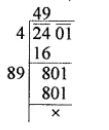Home/Class 8/Maths/

There are 2401 students in a school. P.T. teacher  wants them to stand in rows and columns such that the number of rows is equal  to the number of columns. Find the number of rows.Speed
00:00
02:03## QuestionMathsClass 8

There are 2401 students in a school. P.T. teacher  wants them to stand in rows and columns such that the number of rows is equal  to the number of columns. Find the number of rows.

Total number of rows are 49.
4.64.6## Solution

Let the total number of rows be $$x$$
Then total number of columns be $$x$$
According to Question
$$x \times x=2401$$$$x=49$$ students
Total number of rows are 49.